## Types of Energy Sources:

There are basically two Types of Energy Sources ; voltage source and current source. These are classified as

i) Ideal source and

ii) Practical source.

Let us see the difference between ideal and practical sources.

### Voltage Source

Ideal voltage source is defined as the energy source which gives constant voltage across its terminals irrespective of the current drawn through its terminals. The symbol for ideal voltage source is shown in the Fig. 1.5(a). This is connected to the load as shown in Fig. 1.5(b). At any time the value of voltage at load terminals remains same. This is indicated by V- I characteristics shown in the Fig. 1.5 (c).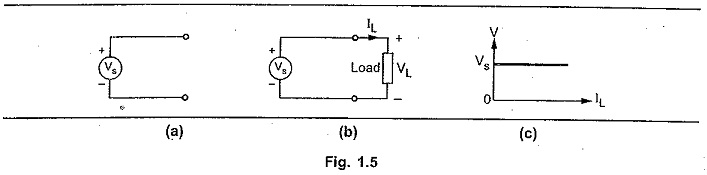But practically, every voltage source has small internal resistance shown in series with voltage source and is represented by Rse as shown in the Fig.1.6.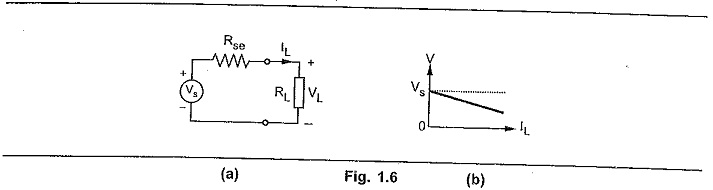Because of the Rse voltage across terminals decreases slightly with increase in current and it is given by expression,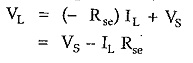For ideal voltage source,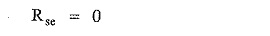Voltage sources are further classified as follows,

#### 1)Time Invariant Sources :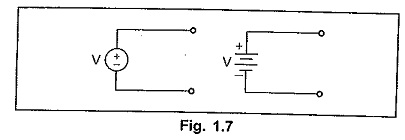The sources in which voltage is not varying with time are known as time invariant voltage sources or D.C. sources. These are denoted by capital letters. Such a source is represented in the Fig. 1.7.

#### 2) Time Variant Sources :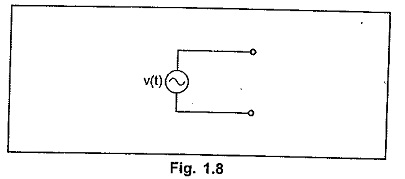The sources in which voltage is varying with time are known as time variant voltage sources or A.C. sources. These are denoted by small letters. This is shown in the Fig. 1.8.

### Current Source

Ideal current source is the source which gives constant current at its terminals irrespective of the voltage appearing across its terminals. The symbol for ideal current source is shown in the Fig. 1.9 (a). This is connected to the load as shown in the Fig. 1.9 (b). At any time, the value of the current flowing through load IL is same i.e. is irrespective of voltage appearing across its terminals. This is explained by V-I characteristics shown in the Fig. 1.9 (c).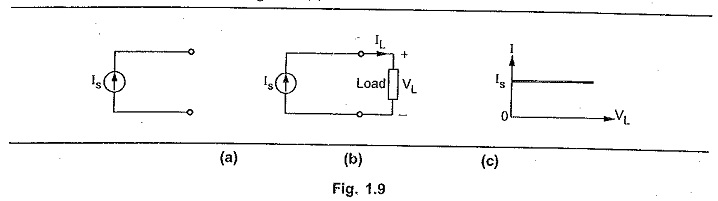But practically, every current source has high internal resistance, shown in parallel with current source and it is represented by Rsh. This is shown in the Fig. 1.10.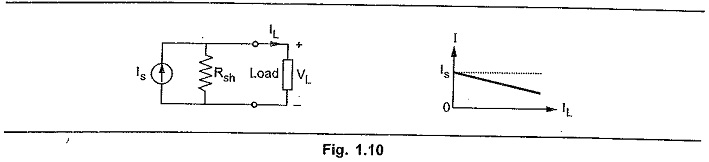Because of Rsh, current through its terminals decreases slightly with increase in voltage at its terminals.

For ideal current source, Rsh = ∞ and generally not shown.

Similar to voltage sources, current sources are classified as follows :

#### 1)Time Invariant Sources :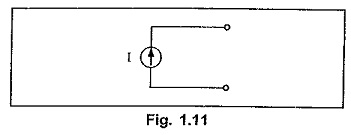The sources in which current is not varying with time are known as time invarient current sources or D.C. sources. These are denoted by capital letters.

#### 2)Time Variant Sources :

The sources in which current is varying with time are known as time variant current sources or A.C. sources. These are denoted by small letters.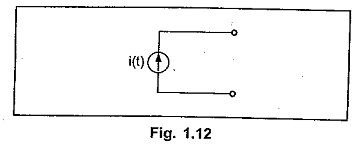Such a source is represented in the Fig. 1.12.

The sources which are discussed above are independent sources because these sources does not depend on other voltages or currents in the network for their value. These are represented by a circle with a polarity of voltage or direction of current indicated inside.

### Dependent sources

Dependent sources are those whose value of source depends on voltage or current in the circuit. Such sources are indicated by diamond as shown in the Fig. 1.13 and further classified as,

##### 1)Voltage Dependent Voltage Source :

It produces a voltage as a function of voltages elsewhere in the given circuit. This is called VDVS. It is shown in the Fig. 1.13 (a).

##### 2)Current Dependent Current Source :

It produces a current as a function of currents elsewhere in the given circuit. This is called CDCS. It is shown in the Fig. 1.13 (b).

##### 3)Current Dependent Voltage Source :

It produces a voltage as a function of current elsewhere in the given circuit. This is called CDVS. It is shown in the Fig. 1.13 (c).

##### 4)Voltage Dependent Current Source :

It produces a current as a function of voltage elsewhere in the given circuit. This is called VDCS. It is shown in the Fig. 1.13 (d).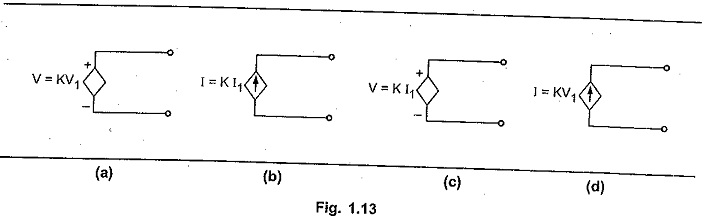K is constant and V1 and I1 are the voltage and current respectively, present elsewhere in the given circuit. The dependent sources are also known as controlled sources.

Scroll to Top